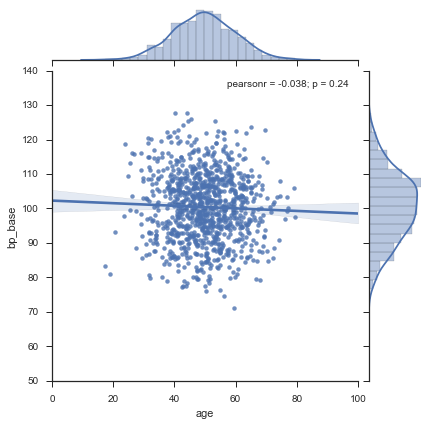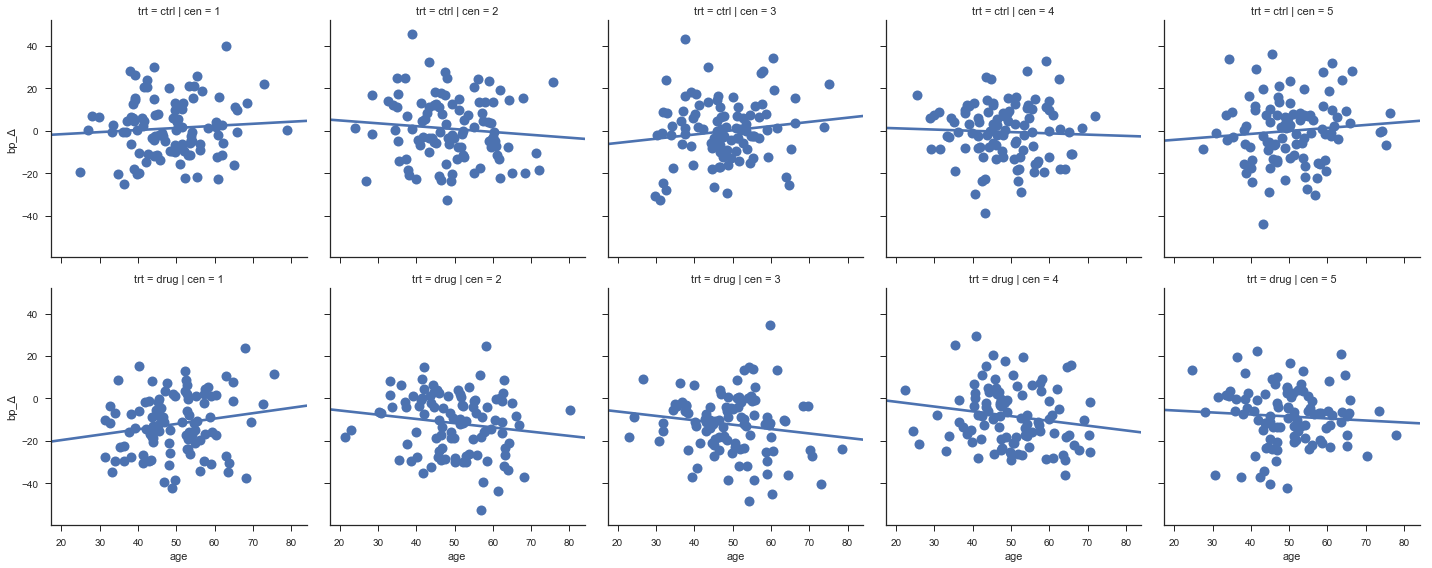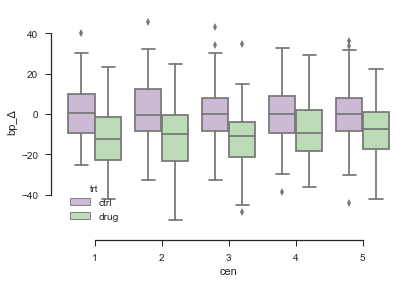.

.

.

.

.

# HealthyNumerics

HealthPoliticsEconomics | Quant Analytics | Numerics

# Meta Analysis 01: Clinical multi center study

Ding-Geng (Din) Chen, Karl E. Peace (2013): Applied Meta-Analysis with R. CRC Press, Taylor & Francis Group, Boca Raton (FL), 321pp. ISBN-10: 1466505990, ISBN-13: 978-1466505995. See also here

The applications in this publication are programmed in R. We do it in Python here. We will describe the cases in a minimalistic way only. For details consult the book please.

### The case

The example in chapter 1 mimics a multi center study of a diastolic blood pressure decreasing drug. The data are generated by ading random noise to a normal distribution. The generated data are concatenated to a data framework which allows for queries, statistical analysis and graphical displays. As a result we get a running workflow for a typical type of analysis.

The example generates for each of the 5 clinical centers the data series for the control group and for the treated group. A series includes the variables age, base pressure, endpoint pressure, difference between them, the number of the clinical center and the type of treament ('drug' = yes, 'ctrl' = no)

### Implementation with Python

#### Import the Python librarys

The add-on libraries to the core of Python are NumPy for the matrix/array data types, and Pandas for data structures and prelimnary data analysis. The statistical package StatsModels is used for the regression model and the analysis of variances. Seaborn is used for the grafics which is based on Matplotlib

import numpy  as np
import pandas as pd
import statsmodels.api as sm
import statsmodels.formula.api as smf
import seaborn as sns
sns.set(style="ticks")
%matplotlib inline


#### Define the functions to generate the data

The first 2 moments - mean and sdev - are used as input to generate a normal distributed data series.

def generateData(nobs,center,treatment,bp_μ, bp_σ,bp_δμ, age_μ, age_σ):
# nobs = n observations
# bp_μ = blood pressure mean
# bp_σ = blood pressure sdev
# bp_δμ= mean change of blood pressure due to drug; = 0 for no drug
# bp_Δ = difference between endpoint and baseline of the clinical trial
bp_base = np.random.normal(bp_μ        , bp_σ, nobs)   # random normal
bp_end  = np.random.normal(bp_μ - bp_δμ, bp_σ, nobs)   # random normal
bp_Δ    = bp_end - bp_base

age     = np.random.normal(age_μ, age_σ, nobs)  # age, random normal
cen     = center*np.ones_like(age,dtype=np.int) # number of the clinical center
trt     = [treatment for t in range(nobs)]      # treatment: with drug or ctrl
index   = np.arange(nobs)                       # data index

myDataStack = {'bp_base': bp_base,'bp_end':bp_end, 'bp_Δ':bp_Δ,
'age':age,'cen':cen,'trt':trt}
return pd.DataFrame(myDataStack,index=index)


#### Set the input data

In this example it is assumed that 5 clinical centers are involved in the multi center study and that bp_δμ (change of mean blood pressure) is the only parameter that varies between the centers. For the control group bp_δμ_ctrl = 0

#------ main --------------------------------
#---- data we keep constant in this example:
nObs=100;  # n observations
trt_ctrl   = 'ctrl'  # control group
trt_drug   = 'drug'  # group with treatment
bp_δμ_ctrl = 0       # change of mean blood pressure in the ctrl-group
bp_μ  = 100          # mean blood pressure
bp_σ  = 10           # sdev blood pressure
age_μ = 50           # mean age
age_σ = 10           # sdev age
#----- data we change to build the series
center1 = 1;  bp_δμ_1 = 10   # clinical center; mean treatment effect
center2 = 2;  bp_δμ_2 = 13
center3 = 3;  bp_δμ_3 = 15
center4 = 4;  bp_δμ_4 =  8
center5 = 5;  bp_δμ_5 = 10


#### Now generate the data series and build a DataFrame

Each clinical center has a data series of the same length for the control group and the treated group. All data are concatenated into 1 data frame.

# series 1
dat4ctrl = generateData(nObs,center1,trt_ctrl,bp_μ,bp_σ,bp_δμ_ctrl,age_μ, age_σ)
dat4drug = generateData(nObs,center1,trt_drug,bp_μ,bp_σ,bp_δμ_1   ,age_μ, age_σ)
d1 = pd.concat([dat4ctrl,dat4drug],ignore_index=True)
# series 2
dat4ctrl = generateData(nObs,center2,trt_ctrl,bp_μ,bp_σ,bp_δμ_ctrl,age_μ, age_σ)
dat4drug = generateData(nObs,center2,trt_drug,bp_μ,bp_σ,bp_δμ_2   ,age_μ, age_σ)
d2 = pd.concat([dat4ctrl,dat4drug],ignore_index=True)
# series 3
dat4ctrl = generateData(nObs,center3,trt_ctrl,bp_μ,bp_σ,bp_δμ_ctrl,age_μ, age_σ)
dat4drug = generateData(nObs,center3,trt_drug,bp_μ,bp_σ,bp_δμ_3   ,age_μ, age_σ)
d3 = pd.concat([dat4ctrl,dat4drug],ignore_index=True)
# series 4
dat4ctrl = generateData(nObs,center4,trt_ctrl,bp_μ,bp_σ,bp_δμ_ctrl,age_μ, age_σ)
dat4drug = generateData(nObs,center4,trt_drug,bp_μ,bp_σ,bp_δμ_4   ,age_μ, age_σ)
d4 = pd.concat([dat4ctrl,dat4drug],ignore_index=True)
# series 5
dat4ctrl = generateData(nObs,center5,trt_ctrl,bp_μ,bp_σ,bp_δμ_ctrl,age_μ, age_σ)
dat4drug = generateData(nObs,center5,trt_drug,bp_μ,bp_σ,bp_δμ_5   ,age_μ, age_σ)
d5 = pd.concat([dat4ctrl,dat4drug],ignore_index=True)
# build 1 DataFrame with all data
dAll = pd.concat([d1,d2,d3,d4,d5],ignore_index=True)


Let's have a look at the head of the first few lines of the data frame:

dAll.head(5)

age bp_base bp_end bp_Δ cen trt
0 60.720858 111.664456 99.437909 -12.226547 1 ctrl
1 36.035688 89.109796 88.445715 -0.664080 1 ctrl
2 38.823806 92.925221 97.549218 4.623998 1 ctrl
3 38.783952 91.520139 104.185235 12.665097 1 ctrl
4 65.327808 93.283620 104.308016 11.024396 1 ctrl

#### Print the statistics of the data

dAll.groupby(['cen', 'trt']).agg(['count','mean','std'])

age bp_base bp_end bp_Δ
count mean std count mean std count mean std count mean std
cen trt
1 ctrl 100 48.467260 10.194531 100 98.567163 11.792550 100 99.793300 9.211106 100 1.226136 13.389781
drug 100 49.628733 9.628830 100 99.935044 10.373236 100 87.838175 9.571521 100 -12.096870 14.092291
2 ctrl 100 49.215135 10.550840 100 98.480676 10.410645 100 99.435871 10.324950 100 0.955195 14.974590
drug 100 50.154245 10.313113 100 98.144471 9.870396 100 86.472468 10.531072 100 -11.672004 14.280153
3 ctrl 100 47.850356 9.693100 100 99.867286 9.946296 100 99.712728 9.894162 100 -0.154558 14.311047
drug 100 49.427241 10.435070 100 98.287170 10.428239 100 86.059913 10.143901 100 -12.227257 14.219824
4 ctrl 100 48.263132 9.648512 100 99.514863 9.863520 100 99.043574 9.933129 100 -0.471289 13.328751
drug 100 49.930536 10.266096 100 99.877485 10.086439 100 91.581340 9.771715 100 -8.296145 13.936570
5 ctrl 100 50.424546 9.768934 100 99.979968 10.454416 100 100.016502 10.191461 100 0.036535 14.902816
drug 100 49.665251 10.003291 100 98.986446 9.906621 100 90.535263 9.178024 100 -8.451183 13.524656

#### Play around with the different types of plots

This first plot contains all data, age vs base blood pressure, treated and untreated over all 5 clinical centers. On the east and the north side of the graph you should recognize the generated normal distributions in the bar plots, whereas the plotted lines are nice looking splines over the bar data only. The plotted line in the graph with y = base blood pressure should be horizontal most of the time and have a value of about 100 beceause this the mean given as input.

Each data series has 100 observations, so 1'000 data points are included in this graph.

sns.jointplot(x="age", y="bp_base", data= dAll, kind="reg");The next graph displays the change of blood pressure vs age for each center. The change of blood pressure should be more negative for the treated groups than for the control group hopefully, beceause this corresponds to the mean given as input.

sns.lmplot(x="age", y="bp_Δ", col="cen", row="trt", data=dAll,
ci=None, palette="muted", size=4,
scatter_kws={"s": 100, "alpha": 1 });The change of blood pressure, displayed for the ctrl-group and the trt-group for each clinical center, is summarized in a box plot.

sns.boxplot(x="cen", y="bp_Δ", hue="trt", data=dAll, palette="PRGn")
sns.despine(offset=10, trim=True)### Data analysis of each center

With these preliminary graphical illustrations, we now proceed to data analysis. We start with the individual center effects. We subset the data from each center to perform an analysis of variance. We consider a treament effect as statistically significant at p-value $$<0.05$$ e.g.

e_c1 = smf.ols(formula='bp_Δ ~ trt', data = dAll[dAll["cen"]==1]).fit()
t_c1 = sm.stats.anova_lm(e_c1, typ=1)
print('Center = 1'); print(t_c1, '\n')

e_c2 = smf.ols(formula='bp_Δ ~ trt', data = dAll[dAll["cen"]==2]).fit()
t_c2 = sm.stats.anova_lm(e_c2, typ=1)
print("Center = 2");  print(t_c2, '\n')

e_c3 = smf.ols(formula='bp_Δ ~ trt', data = dAll[dAll["cen"]==3]).fit()
t_c3 = sm.stats.anova_lm(e_c3, typ=1)
print("Center = 3");  print(t_c3, '\n')

e_c4 = smf.ols(formula='bp_Δ ~ trt', data = dAll[dAll["cen"]==4]).fit()
t_c4 = sm.stats.anova_lm(e_c4, typ=1)
print("Center = 4");  print(t_c4, '\n')

e_c5 = smf.ols(formula='bp_Δ ~ trt', data = dAll[dAll["cen"]==5]).fit()
t_c5 = sm.stats.anova_lm(e_c5, typ=1)
print("Center = 5");  print(t_c5, '\n')

Center = 1
df        sum_sq      mean_sq         F        PR(>F)
trt         1.0   8875.124407  8875.124407  46.97338  8.913676e-11
Residual  198.0  37410.010029   188.939445       NaN           NaN

Center = 2
df        sum_sq      mean_sq          F        PR(>F)
trt         1.0   7972.307055  7972.307055  37.239755  5.404231e-09
Residual  198.0  42387.948440   214.080548        NaN           NaN

Center = 3
df        sum_sq      mean_sq          F        PR(>F)
trt         1.0   7287.503584  7287.503584  35.809995  1.003735e-08
Residual  198.0  40293.937324   203.504734        NaN           NaN

Center = 4
df        sum_sq      mean_sq          F    PR(>F)
trt         1.0   3061.418522  3061.418522  16.464392  0.000071
Residual  198.0  36816.473763   185.941787        NaN       NaN

Center = 5
df        sum_sq      mean_sq          F    PR(>F)
trt         1.0   3602.067373  3602.067373  17.787537  0.000038
Residual  198.0  40096.015373   202.505128        NaN       NaN


### Data Analysis with pooled data from five centers

Remember data generation: Different treatment effects has been assigned to the different clinical centers but treatment has not been related to age. We hope to recognize that in the analysis again of course. We start to fit a model for the blood pressure difference bp_Δ with 3-way interactions among treatment, center and age including the covariates as follows:

$$bp_Δ = a_0\cdot + a_1\cdot TRT + a_2\cdot CEN + a_3\cdot AGE + a_4\cdot TRT\cdot AGR + a_5\cdot TRT \cdot CEN + a_6\cdot CEN \cdot AGE + a_7\cdot TRT \cdot CEN \cdot AGE + \epsilon$$

We perform an analysis of variance on that model and reduce the complexity of the model afterwards motivated by the p-values as a ranking parameter. This gives the following series of models

$$\begin{eqnarray} e_{Pool1} & := & bp_Δ \sim trt*cen*age \\ e_{Pool2} & := & bp_Δ \sim cen + trt*age \\ e_{Pool3} & := & bp_Δ \sim cen + trt + age \\ e_{Pool4} & := & bp_Δ \sim cen + trt \end{eqnarray}$$

Since the increase of the squared sums of the residuals (ssr) is moderate we may conclud that the simplest model is still accurate.

e_pool1 = smf.ols(formula='bp_Δ ~ trt*cen*age', data = dAll).fit()
t_pool1 = sm.stats.anova_lm(e_pool1, typ=1)
print("Pool = all");
print(t_pool1.sort_values("PR(>F)",axis=0)) # output table sorted by PR(>F)

Pool = all
df         sum_sq       mean_sq           F        PR(>F)
trt            1.0   29523.440890  29523.440890  149.114727  4.754130e-32
trt:cen        1.0    1047.327006   1047.327006    5.289759  2.165806e-02
trt:age        1.0     555.765934    555.765934    2.807020  9.416772e-02
trt:cen:age    1.0     370.249746    370.249746    1.870029  1.717815e-01
cen            1.0     235.404079    235.404079    1.188961  2.758041e-01
cen:age        1.0     111.981001    111.981001    0.565585  4.521971e-01
age            1.0      86.740679     86.740679    0.438103  5.081931e-01
Residual     992.0  196407.518177    197.991450         NaN           NaN

e_pool2 = smf.ols(formula='bp_Δ ~ cen + trt*age', data = dAll).fit()
e_pool3 = smf.ols(formula='bp_Δ ~ cen + trt + age', data = dAll).fit()
e_pool4 = smf.ols(formula='bp_Δ ~ cen + trt ', data = dAll).fit()
table = sm.stats.anova_lm(e_pool1, e_pool2, e_pool3, e_pool4, typ=1)
print(table)

   df_resid            ssr  df_diff      ss_diff         F  Pr(>F)
0     992.0  196407.518177      0.0          NaN       NaN     NaN
1     995.0  197953.658419     -3.0 -1546.140243  2.587547     NaN
2     996.0  198479.145133     -1.0  -525.486714  2.638289     NaN
3     997.0  198579.582543     -1.0  -100.437410  0.504262     NaN